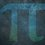# UKMT Special (Problem $14$)

No digit of the positive integer $N$ is prime. However, all the the single-digit primes divide $N$ exactly.

What is the smallest such integer $N$?

[UKMT Hamilton Olympiad $2016$, H$2$]Note by Yajat Shamji
8 months, 2 weeks ago

This discussion board is a place to discuss our Daily Challenges and the math and science related to those challenges. Explanations are more than just a solution — they should explain the steps and thinking strategies that you used to obtain the solution. Comments should further the discussion of math and science.

When posting on Brilliant:

• Use the emojis to react to an explanation, whether you're congratulating a job well done , or just really confused .
• Ask specific questions about the challenge or the steps in somebody's explanation. Well-posed questions can add a lot to the discussion, but posting "I don't understand!" doesn't help anyone.
• Try to contribute something new to the discussion, whether it is an extension, generalization or other idea related to the challenge.

MarkdownAppears as
*italics* or _italics_ italics
**bold** or __bold__ bold
- bulleted- list
• bulleted
• list
1. numbered2. list
1. numbered
2. list
Note: you must add a full line of space before and after lists for them to show up correctly
paragraph 1paragraph 2

paragraph 1

paragraph 2

[example link](https://brilliant.org)example link
> This is a quote
This is a quote
    # I indented these lines
# 4 spaces, and now they show
# up as a code block.

print "hello world"
# I indented these lines
# 4 spaces, and now they show
# up as a code block.

print "hello world"
MathAppears as
Remember to wrap math in $$ ... $$ or $ ... $ to ensure proper formatting.
2 \times 3 $2 \times 3$
2^{34} $2^{34}$
a_{i-1} $a_{i-1}$
\frac{2}{3} $\frac{2}{3}$
\sqrt{2} $\sqrt{2}$
\sum_{i=1}^3 $\sum_{i=1}^3$
\sin \theta $\sin \theta$
\boxed{123} $\boxed{123}$

Sort by:

You have until next Monday, $3:00$pm!

- 8 months, 2 weeks ago

Step 1) The first statement implies that the possible digits of N are 0, 1, 4, 6, 8, and 9.

Step 2) The first statement implies that N is divisible by 2, 3, 5, and 7.

Step 3) Because 2 and 5 divide N exactly, 10 must also divide N. This implies that the last digit of N is 0.

Step 4) Now, lets try listing some positive integer that divisible by 3, 7, and 10.

   210, 420, 630, 840, ...


Notice that 840 is the smallest positive integer that satisfy the statement.

PS : I notice that the third step is not necessary, but it might give you insight on other problem.

- 8 months, 2 weeks ago

Correct, but slightly different method.

- 8 months, 2 weeks ago